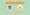# CBSE Class 9 Maths Sample Papers | Download 9th Sample Papers PDFsMathematics is a subject that needs a lot of practice even if the students are well aware of concepts, requires more and more practice to engrave those concepts in your mind. The students must practice CBSE class 9 maths sample papers in order to score higher marks in CBSE Class 9 Maths exam.

The students can find sample papers 2020 which they can download for their reference. It enables the students to score better in their examinations. Solving sample papers is a good way to prepare for the exams as it raises the confidence of the students.

Here we are providing you the complete guide on CBSE Class 9 Maths Sample Papers.

## CBSE Class 9 Maths Sample Papers

CBSE class 9 maths sample papers are the best way of practicing and strengthening the concepts. It assists the students to have a real-time experience of question paper pattern so that they can prepare accordingly. These sample papers assist the students to identify topics that need to be focused more, types of tricky questions, and a lot more.

The students can easily and conveniently download sample papers with solutions to understand the pattern of questions asks in the examination. The students should practice various maths papers of multiple authors for a better understanding of concepts and score good marks.

## What Will You Get In CBSE Class 9 Maths Sample Papers?

9th maths sample papers cover all important concepts and questions from the exam perspective. The students can also download chapter-wise sample papers. The students must learn & practice more on different topics to excel in Maths exams.

• Chapter 1 – Number System
• Chapter 2 – Polynomial
• Chapter 3 – Coordinate Geometry
• Chapter 4 – Linear Equations in Two Variables
• Chapter 5 – Introduction to Euclid’s Geometry
• Chapter 6 – Lines and Angles
• Chapter 7 – Triangles
• Chapter 9 – Areas of Parallelograms
• Chapter 10 – Circles
• Chapter 11 – Constructions
• Chapter 12 – Heron’s Formula
• Chapter 13 – Surface Areas and Volumes
• Chapter 14 – Statistics
• Chapter 15 – Probability

## CBSE Class 9 Maths Sample Papers with Solutions

The students once complete with solving 9th maths sample Question papers can go for the given solutions and compare the answers they have written. This assists the students to prepare for the exams in a better manner and obtain high marks. The students can also download the sample papers SA 1 & SA 2 with solutions PDF for doing practice to score good marks in the exam.

With the help of these sample papers with a solution, the students can improve their weaker sections be it algebra, probability, trigonometry, or geometry. By downloading the PDF, the students can easily access them at any time and anywhere. The students can analyze their exam preparation by going through the marking scheme & answer key and they can work on their weak points accordingly.

## Benefits of CBSE Class 9 Maths Sample Papers

The students who want to achieve high marks in the Class 9 exam must solve all sample question papers. The students come to know the exam pattern and the time to answer every question by solving these sample papers. Here, the students can download the maths sample papers for academic years:

• It provides students the best practice that they can get to caliber the level of their preparation.
• It assists the students to identify their weaker section, removes silly mistakes, focuses more on their strong areas, and get ready for the real exam.
• The students can analyze their knowledge about their maths subject and get more confident about the answers by practicing papers regularly.
• By solving various sample papers of multiple authors help the students to identify their silly mistakes so that they try not to repeat them during the final examination.
• The students can easily download ample papers with solutions in the PDF format and access them anywhere and anytime.

## Other Important Links for CBSE 9th Maths

You should refer below links to know CBSE 9th Maths Exam. Click on the link to access other important information related to the CBSE 9th Maths.

We have covered the detailed guide on CBSE Class 9 Maths Sample Papers PDF. Feel Free to ask any Questions Related to CBSE Class 9 Maths in the comment section below.